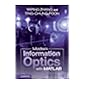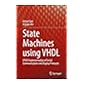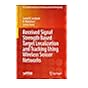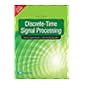Central Library, Indian Institute of Technology Delhi
केंद्रीय पुस्तकालय, भारतीय प्रौद्योगिकी संस्थान दिल्ली

Normal view

# Conceptual digital signal processing with MATLAB

Publication details: Singapore: Springer, 2021Description: xiii, 677 pISBN: 9789811525865UDC classification: 621.391:681.3.06M Summary: This textbook provides an introduction to the study of digital signal processing, employing a top-to-bottom structure to motivate the reader, a graphical approach to the solution of the signal processing mathematics, and extensive use of MATLAB. In contrast to the conventional teaching approach, the book offers a top-down approach which first introduces students to digital filter design, provoking questions about the mathematical tools required. The following chapters provide answers to these questions, introducing signals in the discrete domain, Fourier analysis, filters in the time domain and the Z-transform. The author introduces the mathematics in a conceptual manner with figures to illustrate the physical meaning of the equations involved. Chapter six builds on these concepts and discusses advanced filter design, and chapter seven discusses matters of practical implementation. This book introduces the corresponding MATLAB functions and programs in every chapter with examples, and the final chapter introduces the actual real-time filter from MATLAB. Aimed primarily at undergraduate students in electrical and electronic engineering, this book enables the reader to implement a digital filter using MATLAB. Deliver the conceptual knowledge of digital signal processing with extensive use of the illustrations from practical viewpoint. Also, the digital signal processing is initiated from the digital not from the continuous domain.
List(s) this item appears in: New Arrivals - June | MATLAB
Tags from this library: No tags from this library for this title.
Star ratingsAverage rating: 0.0 (0 votes)
Holdings
Item type Current library Collection Call number Status Date due BarcodeBook Indian Institute of Technology Delhi - Central Library
Central Library
General 621.391:681.3.06M KIM-C (Browse shelf(Opens below)) Available 176663
##### Browsing Indian Institute of Technology Delhi - Central Library shelves, Shelving location: Central Library, Collection: General Close shelf browser (Hides shelf browser)No cover image available621.391 TIC-T Two models of information 621.391:004.438M ZHA-M Modern information optics with MATLAB 621.391:681.3 -MAC Machine learning for future fiber-optic communication systems 621.391:681.3.06M KIM-C Conceptual digital signal processing with MATLAB 621.391:681.3.06V GAZ-S State machines using VHDL FPGA implementation of serial communication and display protocols 621.391.81 JON-R Received signal strength based target localization and tracking using wireless sensor networks 621.391.81 OPP-D Discrete-time signal processing

Includes bibliography and index (p. 673-677)

This textbook provides an introduction to the study of digital signal processing, employing a top-to-bottom structure to motivate the reader, a graphical approach to the solution of the signal processing mathematics, and extensive use of MATLAB. In contrast to the conventional teaching approach, the book offers a top-down approach which first introduces students to digital filter design, provoking questions about the mathematical tools required. The following chapters provide answers to these questions, introducing signals in the discrete domain, Fourier analysis, filters in the time domain and the Z-transform. The author introduces the mathematics in a conceptual manner with figures to illustrate the physical meaning of the equations involved. Chapter six builds on these concepts and discusses advanced filter design, and chapter seven discusses matters of practical implementation. This book introduces the corresponding MATLAB functions and programs in every chapter with examples, and the final chapter introduces the actual real-time filter from MATLAB. Aimed primarily at undergraduate students in electrical and electronic engineering, this book enables the reader to implement a digital filter using MATLAB. Deliver the conceptual knowledge of digital signal processing with extensive use of the illustrations from practical viewpoint. Also, the digital signal processing is initiated from the digital not from the continuous domain.

There are no comments on this title.